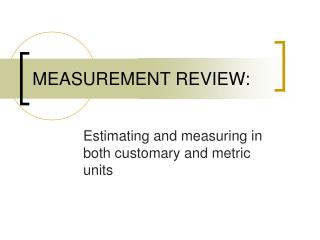DownloadDownload PresentationMEASUREMENT REVIEW:

# MEASUREMENT REVIEW:

Télécharger la présentation## MEASUREMENT REVIEW:

- - - - - - - - - - - - - - - - - - - - - - - - - - - E N D - - - - - - - - - - - - - - - - - - - - - - - - - - -
##### Presentation Transcript

1. MEASUREMENT REVIEW: Estimating and measuring in both customary and metric units

2. Estimating length with non-standard units About how many cubes long is this pencil? Make an estimate.

3. Estimating length with non-standard units This pencil is about 7 cubes long. What was your estimate? How close was your estimate to the actual amount?

4. Estimating length with non-standard units How could you have made a reasonable estimate? Use the length of the cube. Visualize how long 2 cubes would be. 3 cubes. And so on. What else could you have done?

5. Estimating length with non-standard units About how many cubes long is this card? Make an estimate.

6. Estimating length with non-standard units This pencil is about 4 cubes long. What was your estimate? How close was your estimate to the actual amount?

7. Measuring length with customary units Measure the pencil to the nearest ¼ inch. What is your answer? Remember, this ruler has 8 divisions between each inch, so the scale shown is 1/8-inch. There will be two divisions on this scale for each quarter inch.

8. Measuring length with customary units This pencil is about 5-1/2 inches long. The length is five and two-fourths inches. The fraction 2/4 in. is equivalent to 1/2 in.

9. Measuring length with customary units Let’s try another. How long is the card to the nearest 1/4-inch? Be careful! The measurement begins at 1 inch! What is your answer?

10. Measuring length with customary units This card is about 3-1/2 inches long. The measurement began at 1 inch, which means we begin counting at 1 instead of zero. We can also subtract an inch from the amount shown on the scale to get this answer.

11. Measuring length with metric units How long is the paper clip box to the nearest ½ centimeter? We write half of a centimeter as 0.5 cm. So, three and a half cm is written as 3.5 cm. What is your answer?

12. Measuring length with metric units The box is about 7.5 cm long. Each division on this scale is 0.1 cm long, or 1 mm long. Therefore, the box is about 75 mm long.

13. Measuring length with metric units How long is the pencil to the nearest half centimeter? What is your answer?

14. Measuring length with metric units The pencil is about 14.5 cm long. How many mm long is this pencil?

15. Measuring length with metric units The pencil is about 145 mm long. 14 cm is 140 mm. There are 5 more mm to the end of the pencil. That makes 145 mm in all.

16. Summary We hope you enjoyed this review on measuring length. You may email any questions you have to scezell@sikeston.k12.mo.us